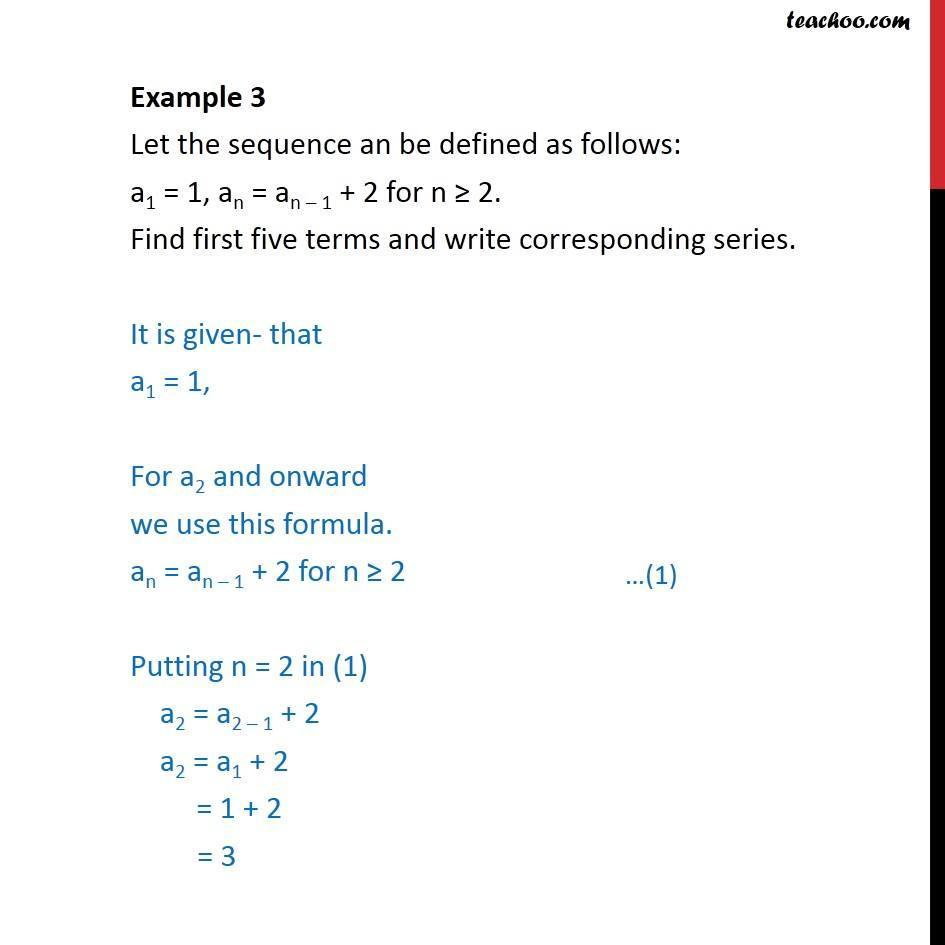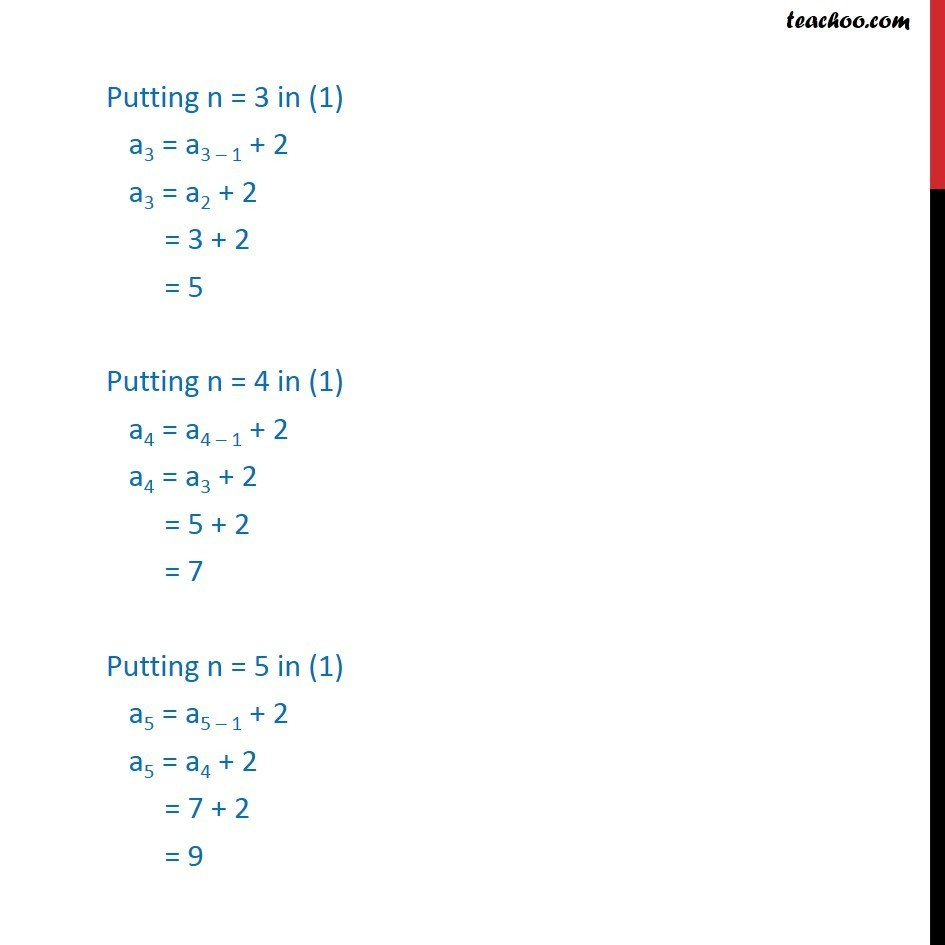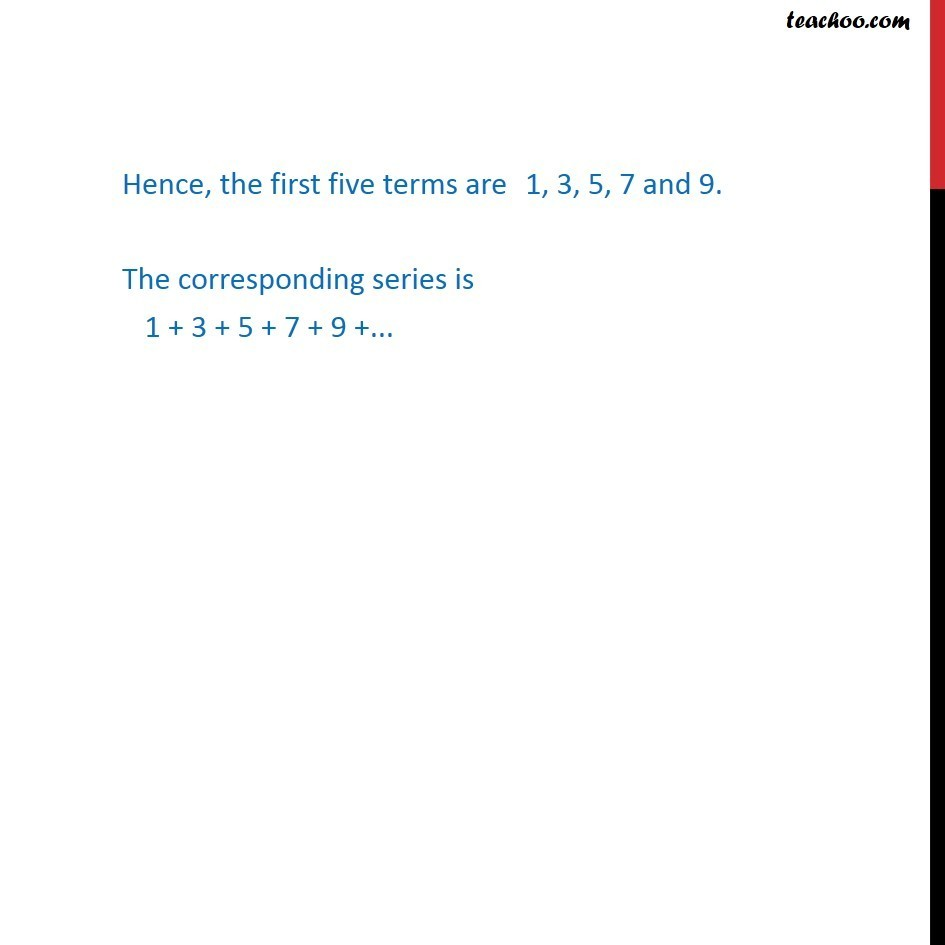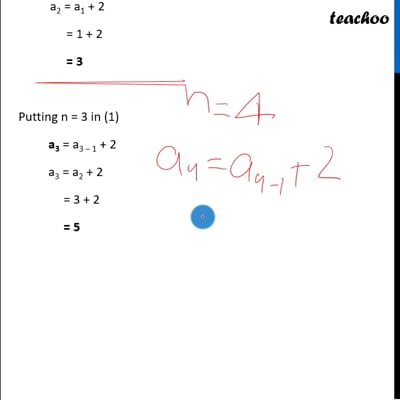Examples

Chapter 8 Class 11 Sequences and Series
Serial order wiseThis video is only available for Teachoo black usersThis video is only available for Teachoo black users

Learn in your speed, with individual attention - Teachoo Maths 1-on-1 Class

### Transcript

Example 3 Let the sequence an be defined as follows: a1 = 1, an = an – 1 + 2 for n ≥ 2. Find first five terms and write corresponding series. It is given- that a1 = 1, For a2 and onward we use this formula. an = an – 1 + 2 for n ≥ 2 Putting n = 2 in (1) a2 = a2 – 1 + 2 a2 = a1 + 2 = 1 + 2 = 3 Putting n = 3 in (1) a3 = a3 – 1 + 2 a3 = a2 + 2 = 3 + 2 = 5 Putting n = 4 in (1) a4 = a4 – 1 + 2 a4 = a3 + 2 = 5 + 2 = 7 Putting n = 5 in (1) a5 = a5 – 1 + 2 a5 = a4 + 2 = 7 + 2 = 9 Hence, the first five terms are 1, 3, 5, 7 and 9. The corresponding series is 1 + 3 + 5 + 7 + 9 +...